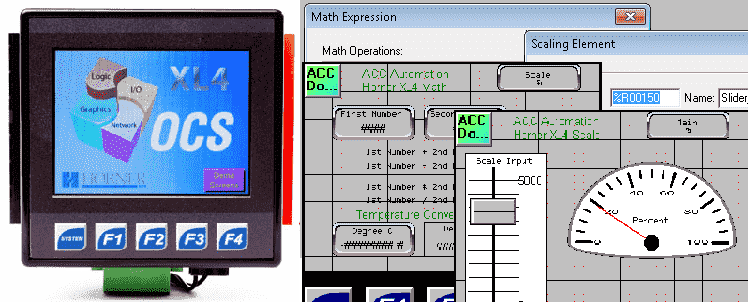Horner XL4 Math Instructions

Math instructions in the Horner APG XL4 OCS all-in-one controller are broken down into Basic Math Operations and Advanced Math Operations.
Basic Math Operations include the following:
Math Equation Element, Add, Subtract, Multiply, Divide, Modulo, Square Root, Absolute Value
Advanced Math Operations include the following:
Power of X, Base 10 Logarithm, Power of e, Natural Logarithm, Sine, Cosine, Tangent, Inverse Sine, Inverse Cosine, Inverse Tangent, Convert to Radians, Convert to Degrees, Scale

We will be looking at the expression and scaling math functions.

Previously we discussed the following in our Horner XL4 Series:
System Hardware
Installing the Software Cscape
Cscape Software Setup
Establishing Communication
Numbering System and Addressing
User Interface
Timers and Counters
Compare and Move Instructions

Math Expression
The math equation element will allow us to write complex math equations that will be solved when the instruction is executed. It operates on a 16 or 32 bit signed integer or 32 bit real numbers. You cannot mix these numbers in the equation instruction.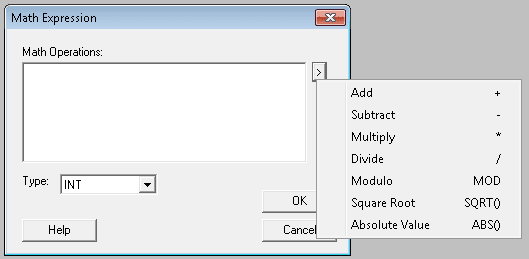To configure the math equation it must be in the following format.
[result] = [equation]
When the instruction is activated, or on the equation is solved. If a math error occurs (divide by zero), the element is off and the value placed in the result is invalid.
Here is some logic in the PLC ladder logic diagram example.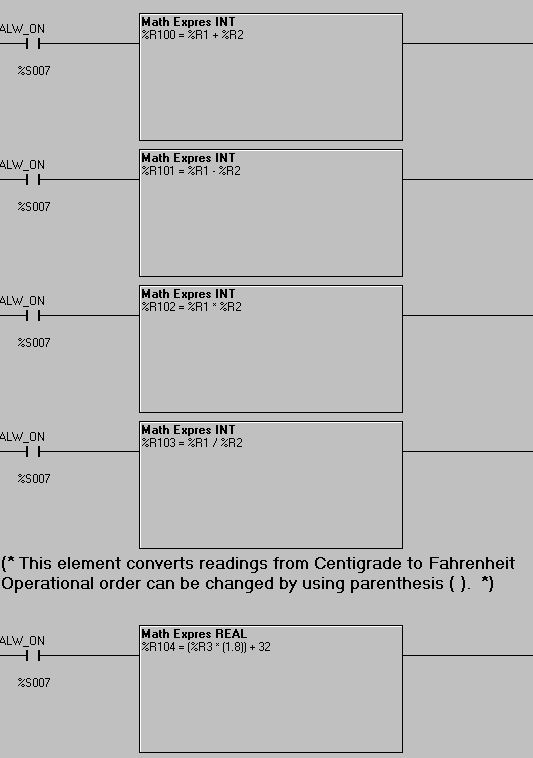%R100 = %R1 + %R2 – Adding the numbers
%R100 = %R1 – %R2 – Subtracting the numbers
%R100 = %R1 * %R2 – Multiplying the numbers
%R100 = %R1 / %R2 – Dividing the numbers
The last rung in the ladder above will convert Centigrade to Fahrenheit. You will notice that the brackets can be nested as much as you like. The limit is 80 characters for the equation.
%R104 = (%R3 * (1.8)) + 32
Real numbers were used because of the decimal point used in the equation. If I had used 9/5 instead of 1.8 then my equation would not have been accurate when using the INT and DINT values.
Here is our Horner APG screen for the math expression instruction.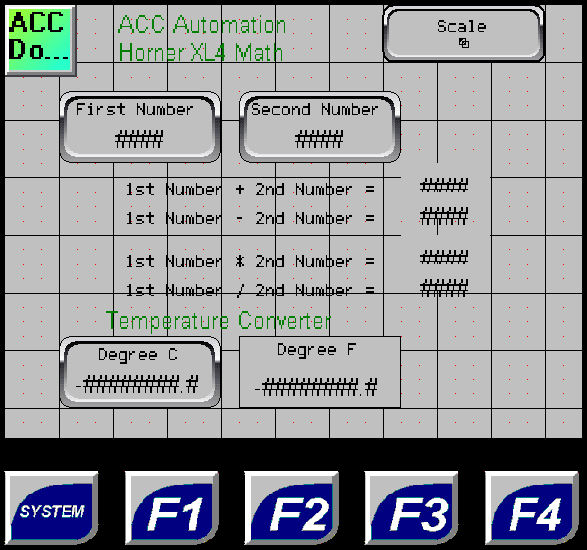See this logic in action by watching the YouTube video below.

Scaling
The scaling math function is often used to convert an analog value into something that is more meaning full to the operator.
Note: Scaling will only work on INT, Floating Point (Real) and Double Floating Point (LReal) numbers.
Let’s look at an example.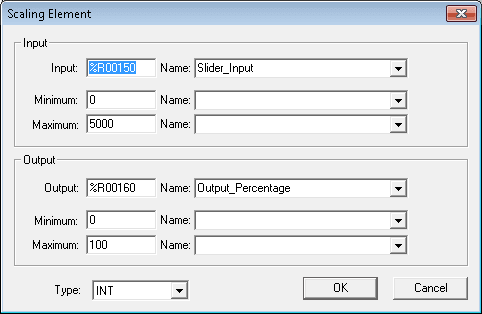We are going to use a slider input on the screen to go from 0 to 5000. This input is coming from register %R00150. The scale instruction will take this value from 0 to 5000 and give me a percent. %R00160 will be the output that is scaled from 0 to 100.
Here is the PLC ladder logic diagram example for the instruction.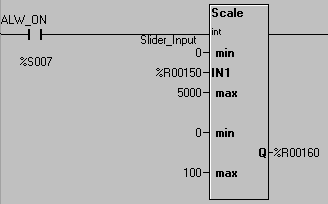The system bit %S007 is the always on bit that will always execute this scale instruction.
Here is our Horner APG screen for the scale instruction.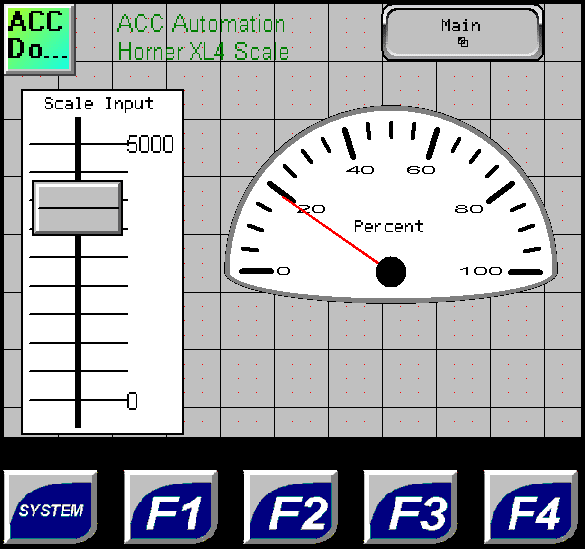See this logic in action by watching the YouTube video below.

You can download this application here. It will contain all of the screens and logic programming.

All of the documentation for the XL4 can be downloaded at the following URL:
http://www.horner-apg.com/xl4

Next time we will look at program control instructions.

Watch on YouTube : Horner XL4 Math Instructions
If you have any questions or need further information please contact me.
Thank you,
Garry

If you’re like most of my readers, you’re committed to learning about technology. Numbering systems used in PLC’s are not difficult to learn and understand. We will walk through the numbering systems used in PLCs. This includes Bits, Decimal, Hexadecimal, ASCII and Floating Point.

To get this free article, subscribe to my free email newsletter.

Use the information to inform other people how numbering systems work. Sign up now.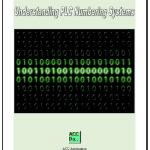The ‘Robust Data Logging for Free’ eBook is also available as a free download. The link is included when you subscribe to ACC Automation.